StatLect

# Random matrix

A random matrix is a matrix whose entries are random variables.## Associated random vector

We can analyze a random matrix by studying the characteristics of its associated random vector, which is obtained by stacking the columns of the matrix.

For example, given a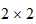random matrixwe can study its associated vectorization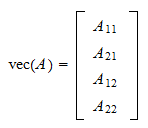which is a random vector.

Another example is a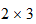random matrixwhose vectorization is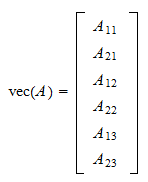Thus, all the theory developed for random vectors applies to random matrices.

For example, a random matrix is said to be:

## Random matrix theory

A flourishing area of modern mathematics, called random matrix theory, studies the distributional properties of some characteristics of random matrices such as their eigenvalues and determinants.

Important results in random matrix theory are:

1. the Wigner semicircle law (distribution of the eigenvalues of a symmetric matrix);

2. the Wigner surmise (distribution of the spaces between the eigenvalues);

3. the Circular law (distribution of the eigenvalues of a non-symmetric matrix);

4. the Tracy-Widom law (distribution of the largest eigenvalue of a matrix);

5. the Marchenko-Pastur law (distribution of the singular values of a matrix).

This blog post provides an elementary description of some of these results.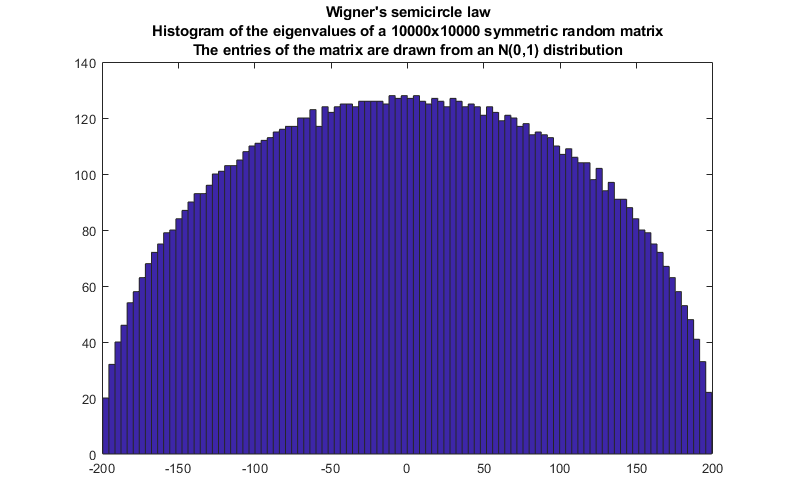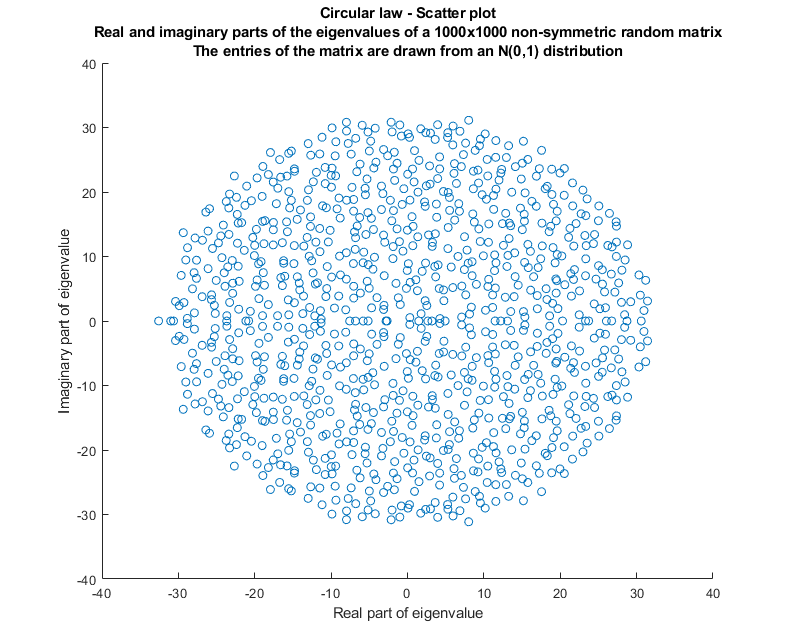## The most important matrix distribution

Probably, the most important random matrix distribution is the Wishart distribution. It is often used in probability and statistics and it has many interesting connections with other distributions such as the Gamma and the multivariate normal.

## Other matrix distributions

Several other matrix distributions are used in mathematical and statistical applications.

Here is a list.

1. Generalizations of vector distributions:

2. Gaussian distributions:

3. Circular distributions:

Recent studies analyze also the properties of some discrete matrix distributions.

## More details

Curious to know more about random matrix theory? Here are some suggestions:

## Keep reading the glossary

Previous entry: Probability space

Next entry: Realization of a random variable